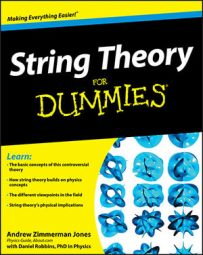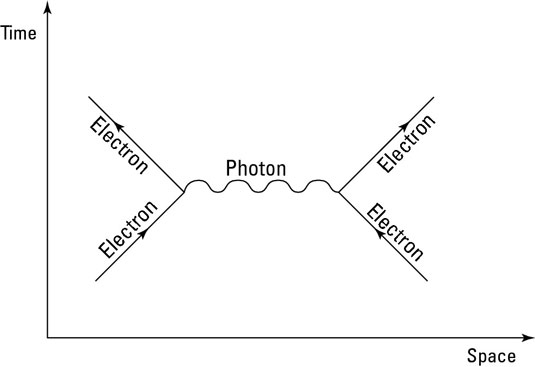##### String Theory For DummiesPhysicists have found a large number of particles, and one thing that proves useful is that they can be broken down into categories based on their properties. Physicists have found a lot of ways to do this, but here are some of the most relevant categories to string theory.

According to quantum mechanics, particles have a property known as spin. This isn’t an actual motion of the particle, but in a quantum mechanical sense, it means that the particle always interacts with other particles as if it’s rotating in a certain way.

In quantum physics, spin has a numerical value that can be either an integer (0, 1, 2, and so on) or half-integer (1/2, 3/2, and so on). Particles that have an integer spin are called bosons, while particles that have half-integer spin are called fermions.

## Particles of force: Bosons

Bosons, named after Satyendra Nath Bose, are particles that have an integer value of quantum spin. The bosons that are known act as carriers of forces in quantum field theory, as the photon does in this figure. The Standard Model of particle physics predicts five fundamental bosons, four of which have been observed:

• Photon

• Gluon (there are eight types of gluons)

• Z boson

• W boson (actually two particles — the W+ and W bosons)

• Higgs boson (this one hasn’t been found yet)In addition, many physicists believe that there probably exists a boson called the graviton, which is related to gravity. Composite bosons can also exist; these are formed by combining together an even number of different fermions.

For example, a carbon-12 atom contains six protons and six neutrons, all of which are fermions. The nucleus of a carbon-12 atom is, therefore, a composite boson. Mesons, on the other hand, are particles made up of exactly two quarks, so they are also composite bosons.

## Particles of matter: Fermions

Fermions, named after Enrico Fermi, are particles that have a half-integer value of quantum spin. Unlike bosons, they obey the Pauli exclusion principle, which means that multiple fermions can’t exist in the same quantum state.

While bosons are seen as mediating the forces of nature, fermions are particles that are a bit more “solid” and are what we tend to think of matter particles. Quarks are fermions.

In addition to quarks, there is a second family of fermions called leptons. Leptons are elementary particles that can’t (so far as scientists know) be broken down into smaller particles. The electron is a lepton, but the Standard Model of particle physics tells us that there are actually three generations of particles, each heavier than the last.

The three generations of particles were predicted by theoretical considerations before they were discovered by experiment, an excellent example of how theory can precede experiment in quantum field theory.

Also within each generation of particles are two flavors of quarks. This table shows the 12 types of fundamental fermions, all of which have been observed. The numbers shown are the masses, in terms of energy, for each of the known particles. (Neutrinos have virtually, but not exactly, zero mass.)

Elementary Particle Families for Fermions
Quarks Leptons
First Generation Up Quark
3 MeV
Down Quark
7 MeV
Electron Neutrino Electron
0.5 MeV
Second Generation Charm Quark
1.2 GeV
Strange Quark
120 MeV
Muon Neutrino Muon
106 MeV
Third Generation Top Quark
174 GeV
Bottom Quark
4.3 GeV
Tau Neutrino Tau
1.8 GeV

There are also, of course, composite fermions, made when an odd number of fermions combine to create a new particle, such as how protons and neutrons are formed by combining quarks.